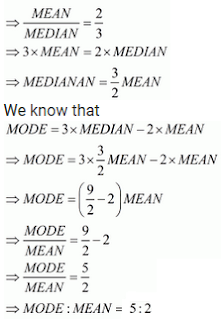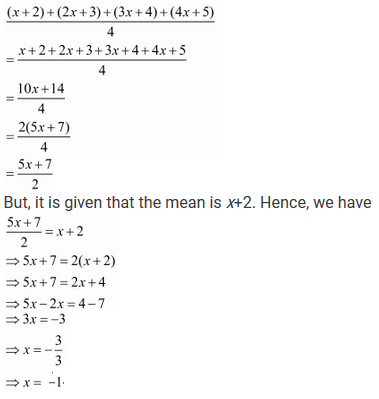## Chapter 24 Measure of Central Tendency R.D. Sharma Solutions for Class 9th MCQ's

Multiple Choice Questions

1. If the ratio of mean and median of a certain data is 2:3, then find the ratio of its mode and mean.

Solution

Given that the ratio of mean and median of a certain data is 2:3. That is,2. If the ratio of mode and median of a certain data is 6 : 5, then find the ratio of its mean and median.

Solution

Given that the ratio of mode and median of a certain data is 6:5. That is,3. If the mean of x + 2, 2x + 3, 3x + 4, 4x + 5 is x + 2, find x .

Solution

The given data is x+2, 2x+3, 3x+4, 4x+5. They are four in numbers.
The mean is4. The arithmetic mean and mode of a data are 24 and 12 respectively, then find the median of the data.

Solution

If the difference of mode and median of a data is 24, then find the difference of median and mean.
Given that the arithmetic mean and mode of a data are 24 and 12 respectively. That is,
Mean = 24
Mode = 12
We have to find median.
We know that,
Mode = 3 × Median - 2 × Mean
⇒ 12 = 3 × Median - 2 × 24
⇒ 3 × Median = 12 + (2 × 24)
⇒ 3 × Median = 12 + 48
⇒ 3 × Median = 60
⇒ Median = 60/3
⇒ Median = 20

5. If the difference of mode and median of a data is 24, then find the difference of median and mean.

Solution

Given that the difference of mode and median of a data is 24. That is,
Mode - Median = 24
⇒ Mode = Median + 24
We have to find the difference between median and mean
We know that,
Mode = 3 × Median - 2 × Mean
⇒ Median + 24 = × Median - 2 × Mean
⇒ 24 = 3 × Median - Median - 2 × Mean
⇒ 24 = 2 × Median - 2 × Mean
⇒ 2 × Median - 2 × Mean = 24
⇒ 2(Median - Mean) = 24
⇒ Median - Mean = 24/2
⇒ Median - Mean = 12

6. If the median of scores x/2, x/3, x/4, x/5 and x/6 (where x > 0) is 6, then find the value of x/6.

Solution7. If the mean of 2, 4, 6, 8, x, y is 5, then find the value of x + y.

Solution

The given data is 2,4,6,8,x,y. They are 6 in numbers.
The mean is
2+4+6+8+x+y/6 = 20+x+y/6
But, it is given that the mean is 5. Hence, we have
20+x+y/6 = 5
⇒ 20+x+y = 30
⇒ x+y = 30 - 20
⇒ x+y = 10

8. If the mode of scores 3, 4, 3, 5, 4, 6, 6, x is 4, find the value of x.

Solution

The given data is 3,4,5,4,6,6,x.

The mode is the value which occur maximum number of times, that is, the mode has maximum frequency. If the maximum frequency occurs for more than 1 value, then the number of mode is more than 1 and is not unique.

Here it is given that the mode is 4. So, x must be 4, otherwise it contradicts that the mode is 4. Hence x = 4

9. If the median of 33, 28, 20, 25, 34, x is 29, find the maximum possible value of x.

Solution10. If the median of the scores 1, 2, x, 4, 5 (where 1 < 2 < x < 4 < 5) is 3, then find the mean of the scores.

Solution

The given data is 1, 2, x, 4 and 5. Since 1<2<x<4<5, the given data is already in ascending order.
Here, the number of observation n = 5, which is an odd number.
Hence, the median is